Mobile QR Code1. (Division of National Supercomputing R&D, Korea Institute of Science and Technology Information, Daejeon 34141, Korea)

Metal halide perovskites, realistically sized nanostructures, band gap engineering, halide phase separation, tight-binding simulations

## I. INTRODUCTION

Implementation of optoelectronic devices using metal halide perovskite (MHP) materials has been keen interest to researchers ever since the successful demonstration of efficient light absorbers for photovoltaic cells (1,2). Light-emitting diode (LED) has been particularly a focal target application of MHPs (3-5) due to promising properties such as narrow emission bands and a flexible tunability of band gap energies (6-8). Upon the first demonstration of room-temperature working MHP LEDs (3), their quantum efficiencies have increased exceeding 20% these days (5).

Precise control of band gap energies is critical to design qualified LEDs. As the emission spectra of MHP samples heavily depend on their confinement structures and halide types (6,7), modeling-based prediction including realistic conditions of MHP samples, e.g. lithographically feasible sizes and uncertainty of atomic positions in alloys, etc., are thus important for procurement of critical design factors, which is in principle hard to be covered by experimental efforts due to the wide range of control variables. Band gap engineering with halide mixtures, particularly, is the focal research topic these days as it has opened the feasibility of fine control of emission wavelengths in a visible spectrum (9,10). While MHP band structures have been theoretically studied with the state-of-the-art Density Function Theory (DFT) simulations (11-13), focuses are limited to bulks or ultra-thin layers that are simulated using extremely tiny supercells (including < 100 atoms) with periodic boundary conditions.

In this work, we expand the simulation scopes of MHP samples to confined structures in over 10-nm scales. With an atomistic tight-binding (TB) model that uses parameter sets fitted to match bulk band structures known by either experiments or DFT studies (14,15), the effects of size and composition engineering of band gaps are understood for MHP nanostructures including up to ~100K atoms. Using methylammonium lead iodide and bromide (MAPbI$_{3}$/Br$_{3}$) as target materials, we examine the tunability of band gaps with size/composition engineering, presenting a complete map of band gap energies that are theoretically achievable. Also, the well-known red-shift issue stemming from phase separation in halide mixtures (7,8,16,17), is investigated and strategies to reduce the red-shift are proposed. Sound connections to recent experimental studies on bulk MHPs are also presented to validate our approach, establishing a modeling framework for realistically sized, confined MHP structures.

## II. METHODOLOGY

Four types of supercells are considered to understand confinement effects on band gap energies, e.g. cubic (box) and sphere quantum dots (QDs), square nanowires (NWs), and quantum wells (QWs), as described in Fig. 1. All the supercells are assumed to be in a cubic phase according to the crystalline characteristics known at T=300K (18,19). Confinement sizes are varied within 3-30 unitcells (ucs), so the largest supercell of modeling targets has 1.08x10$^{5}$ atoms. An 8-band $sp^3$ TB model is employed to represent an atom in electronic structures with spin-orbit coupling. The TB parameter sets reported by Boyer-Richard et al. and Ashhab et al. (20,21) are utilized to model of MAPbI$_{3}$ and MAPbBr$_{3}$, respectively, where the two previous works focused on bulks (20) or small supercells consisting up to 8$^{3}$ cubic unitcells (21). MAPbI$_{3}$ and MAPbBr$_{3}$ bulk band gap energy and band offset computed with our parameters are presented in Table 1.

Table 1. Bulk MAPbI$_{3}$/Br$_{3}$ band gap energies and offsets that are obtained with simulations using an 8-band $sp^3$ TB model

 MHP type Band gap energy (eV) Offset w.r.t. MAPbI$_3$ (eV) Conduction band Valence band MAPbI$_3$ 1.60 - - MAPbBr$_3$ 2.30 +0.01 -0.69

Fig. 1. Simulated supercell structures. Confinement size (L) of 3-30 unitcells (ucs) are taken into consideration in this work.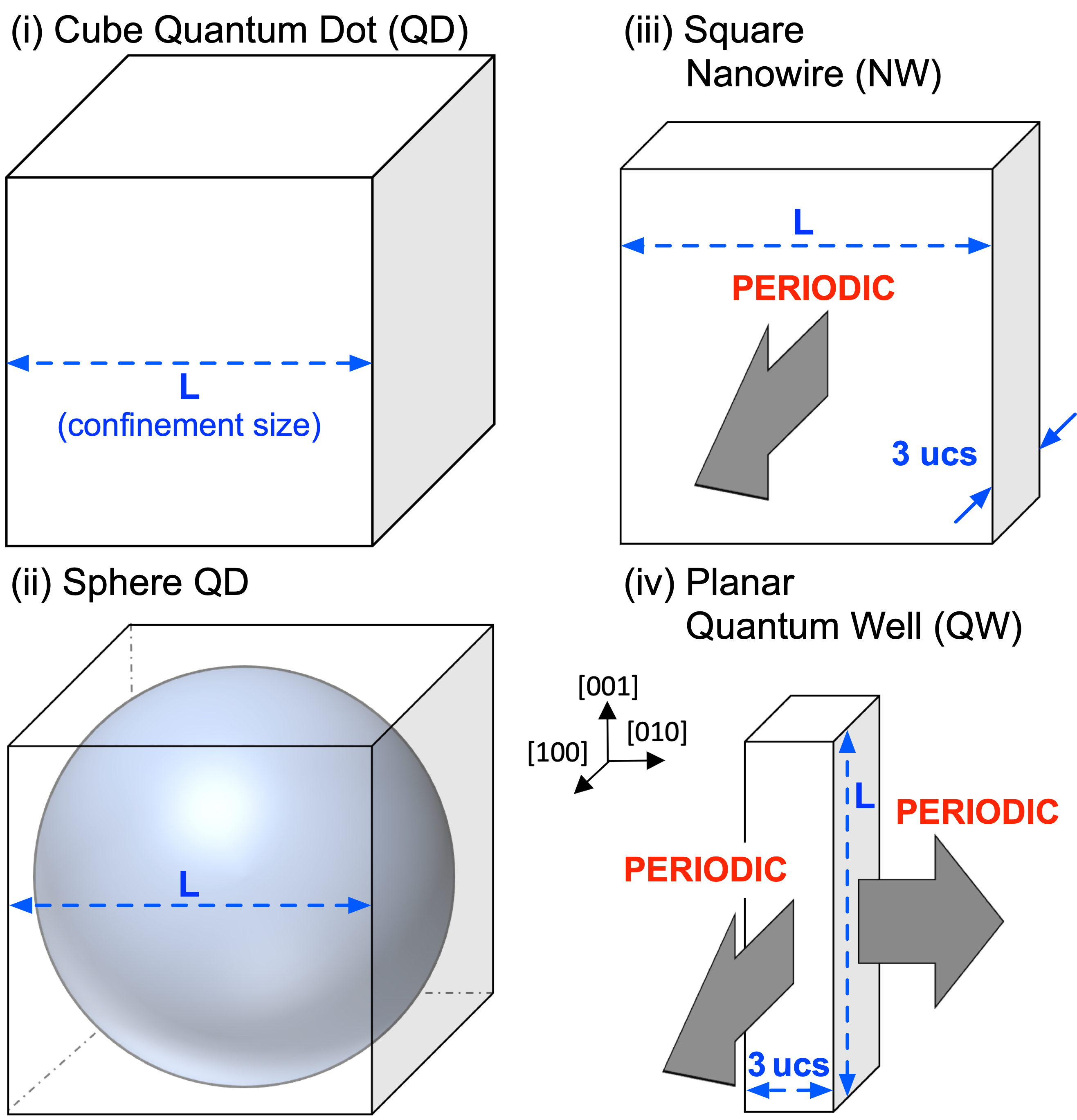TB simulations of electronic structures involve a large-scale diagonalization problem, as the size of Hamiltonian matrices are linearly proportional to the number of atoms residing in supercells to be modelled. Workloads of these diagonalizations are processed with our in-house solvers, which has demonstrated the capability to solve electronic structures for supercells consisting of up to 4 billion atoms with a parallel computing (22-24). The NURION high performance computing cluster (25) is extensively utilized for all the simulations.

## III. RESULTS AND DISCUSSION

### 1. Band Gap Energies with Homogeneous Halides

The feasibility of size and shape engineering as control factors for band gap energies are examined for supercells shown in Fig. 1. Simulation results are summarized in Fig. 2, where band gap energies are converted to wavelengths (in a nm unit) for convenience of discussion with respect to the color spectrum that is included as an inset of Fig. 2. At a room temperature, band gap energy of bulk MAPbI$_{3}$ and MAPbBr$_{3}$ are about 774 nm and 540~nm, respectively. As confinement effects prevail with reduction of supercell sizes, however, energies start to decrease. In overall, our results observe that band gap energies of 375-774 nm and 313-539 nm are theoretically achievable with MAPbI$_{3}$ and MAPbBr$_{3}$ supercells simulated in this work. Confinement effects on band gap energies are much larger in MAPbI$_{3}$ than MAPbPb$_{3}$, so MAPbI$_{3}$ can be considered for designs of green light sources with 1D confinement (QWs), and blue-to-violet sources with 2/3D confinement (NWs/QDs). MAPbBr$_{3}$ is also suitable for green-to-violet light sources, but is not feasible to cover higher wavelengths since the maximally achievable value is the bulk value (540 nm).

Fig. 2. Band gap energies of various nanostructures calculated for MAPbI3 (top) and MAPbBr$_3$ (bottom). Energies are shown as wavelength (nm) for easier connection to the color spectrum.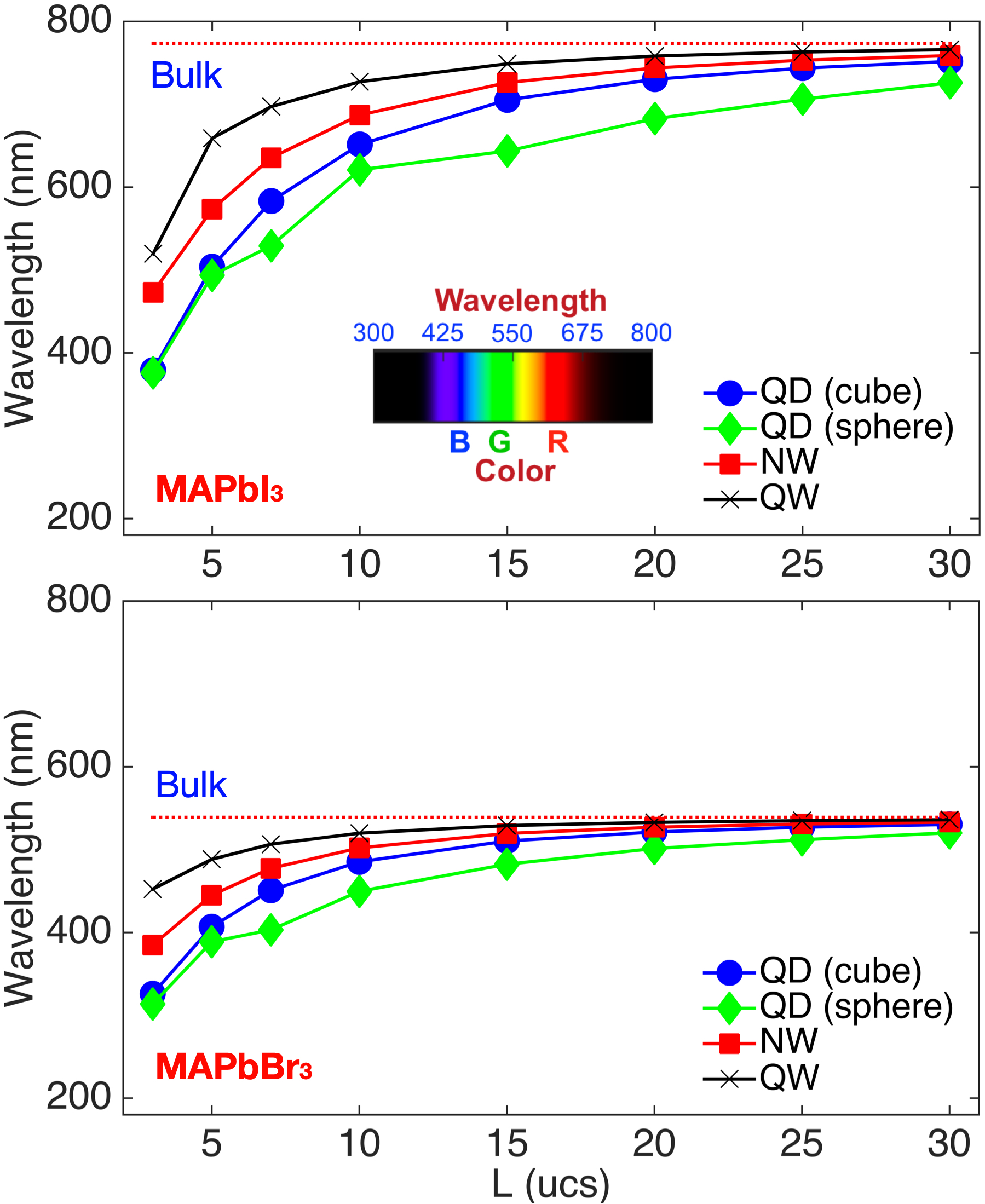Another interesting point that is worthy of discussion is when confined supercells would lose bulk characteristic, since the answer can be used as a practical initial guideline to those who want to reduce the size of absorption layers with no changes in band gap energies known for bulks. To understand this, deviation of band gap energies against the bulk value is calculated for all the supercells. Results are plotted in Fig. 3 as a function of confinement sizes, where 20 nm (a ballpark value of emission band demonstrated for MAPbI$_{\mathrm{x}}$Br3-x bulk samples by Yoon et al. (8)) is taken as a criterion to determine whether the supercells have bulk characteristic. Here, results reveal that bulk characteristic is lost even in supercells of confinement sizes larger than 10nm. Sphere QDs, which would be most severely affected by quantum confinement than other types of supercells at the same confinement size, already experience a deviation of 18.6~nm (MAPbBr$_{3}$) and 47.6 nm (MAPbI$_{3}$) even when the confinement size of 30 ucs (18-19 nm). Confinement effects of cube QDs and NWs are weaker than sphere QDs, but in the case of MAPbBr$_{3}$ that generally exhibits smaller shift of band gap energies compared to MAPbI$_{3}$, NWs and cube QDs lose their bulk characteristics at a confinement size of 15 ucs (9 nm) and 19 ucs (11 nm), respectively.

Fig. 3. Deviation of band gap energies calculated for confined supercells with respect to the bulk value. 20 nm, a ballpark value of emission band known for MAPbI$_{\mathrm{x}}$Br$_{\mathrm{3-x}}$ bulk samples, is used as a criterion to determine whether a supercell is a bulk.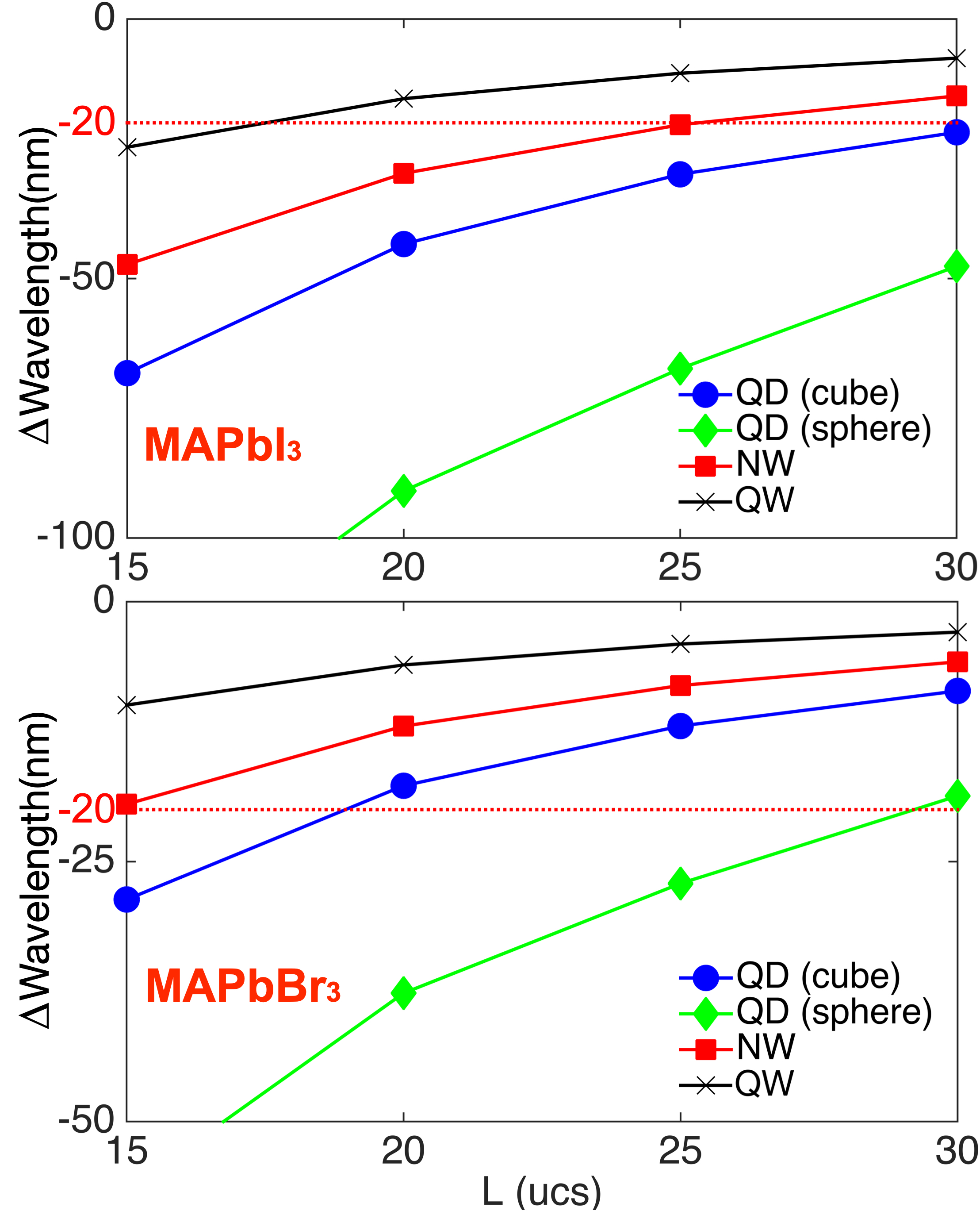### 2. Band Gap Energies with Mixed Halides

As known by previous studies for bulk MHP samples (9,10), composition ratio in mixed halides can be utilized as a control factor of band gap energies. The random nature of halide distributions, however, would drive unexpectedness of band gap energies and is not easy to be avoided during lithographical processes. To explore the effects of random distribution on band gap energies in confined structures of halide mixtures, electronic structures of MAPbI$_{\mathrm{x}}$Br$_{\mathrm{3-x}}$ cube QDs are simulated. For the four composition ratios (20, 40, 60, 80% of Iodide), 100 cases of uniform distributions are considered per each composition ratio. In Fig. 4, results of selective confinement sizes (05, 10, 15, 30 ucs) are shown with red curves, where the error bar plotted on top of each red curve indicates energy fluctuation stemming from 100 random distributions of I and Br atoms. Here, our results reveal that band gap fluctuations due to the randomness in halide distributions increases as confinement sizes reduce, so, when the confinement size is 5 ucs, band gap energies fluctuates up to on the order of the latest reported emission band (~20nm). In supercells of larger confinement sizes, however, effects of random halide placement on band gap energies are not remarkable, as long as we do not consider the extreme cases where halide atoms are placed such that they cannot be considered to be uniformly distributed.

Fig. 4. Band gap energy simulated for MAPbI$_{\mathrm{x}}$Br$_{\mathrm{3-x}}$ cube QDs. From the top left, results of confinement size of 5, 10, 15, and 30 ucs are shown in a clockwise manner. Conceptual Illustration of uniform and segregated distribution of halide atoms are also presented in bottom.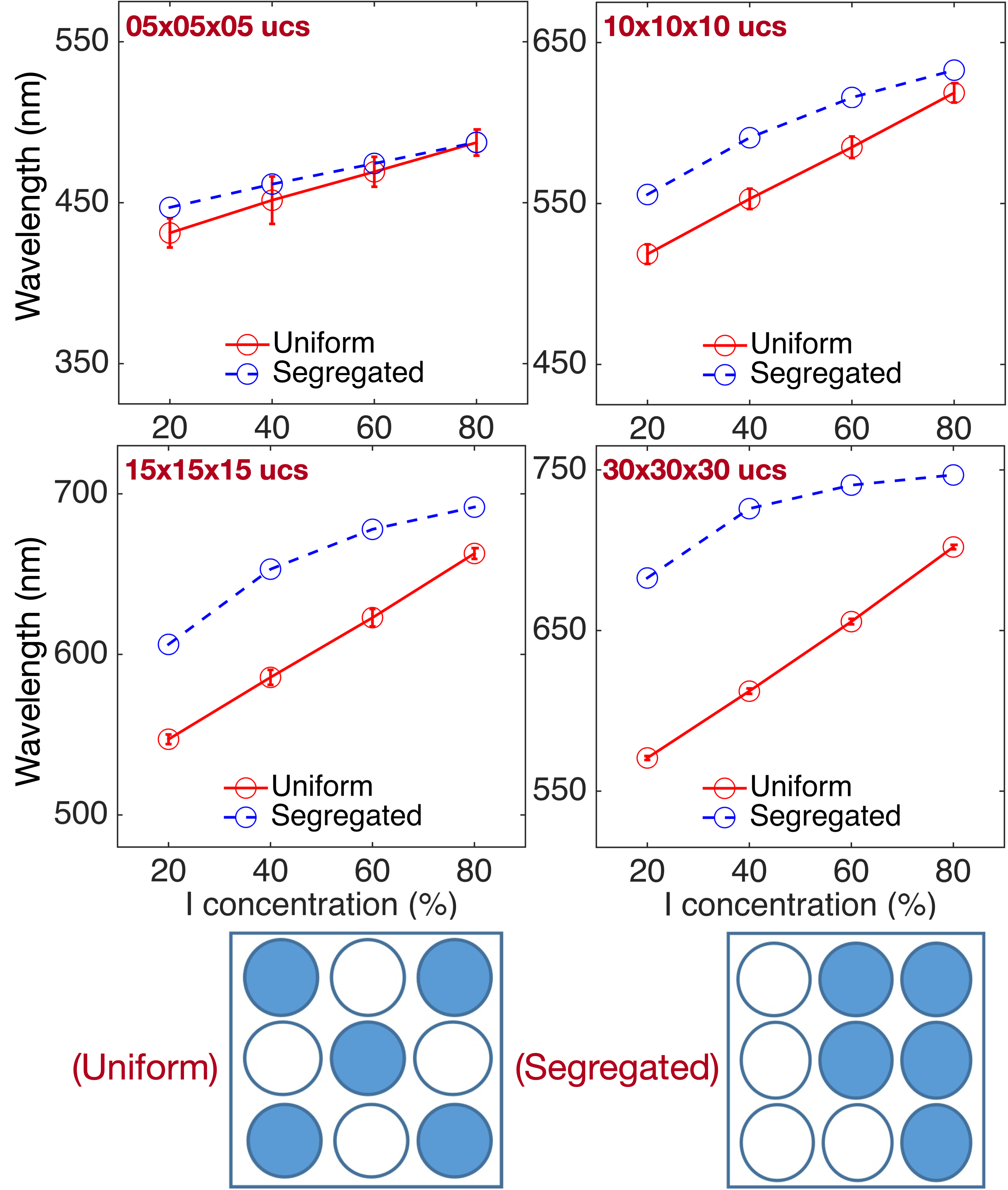Because simulations of 100 random cases would not be sufficient enough to capture the above-mentioned extreme cases of halide distributions, we simulated one more cases by artificially arranging halide positions such that I and Br are completely segregated as illustrated in the right bottom in Fig. 4, and results are presented with blue dotted curves in Fig. 4. In reality, when the sample are exposed to light, I and Br atoms in halide mixtures are strongly segregated, causing a non-negligible red-shift (9,10). The blue dotted curves in Fig. 4, compared to the red curves (i.e. results of uniform distributions), also support this red-shift against the mean value of uniformly distributed cases at the same confinement size and composition ratio. One interesting feature that can be found here, is that the red-shift reduces as the fraction of I atoms (i.e., halides of lower electro-negativity) increases, regardless of confinement sizes. So, at a confinement size of 30 ucs, the red-shift with 20% of I is about 20% of the average of uniformly distributed cases, while it can be reduced to 6% by including 80% of I. The situation is still same in QDs of a confinement size of 5 ucs, so 4% of the red-shift is observed with 20% of I, and this red-shift reduces to 0.04% with 80% of I. It should be noted that confinement sizes themselves also have non-negligible connections to the red-shift. When we focus on the cases where 20% of I is included, for example, the red-shift is about 20% at a confinement size of 30 ucs, but it reduces to 11%, 7%, and 4% at a confinement size of 15, 10, and 5 ucs, respectively.

Halide segregation is a well-known phenomenon caused by phase separation mainly stemming from lights (photo-irradiation). While there are studies that focus on physical origins of light-induced phase separation (7,8), remarkable solutions to get rid of this issue is not available yet. In this situation, we note the messages in the above paragraph are critical, since they reveal the potential for huge reduction of the red-shift through size and composition engineering, which eventually accelerate the feasibility of stable light source designs with halide mixtures.

### 3. Validation: Connections to Experiments

As a final point of discussion, we validate our modeling approach, particularly by analyzing simulation results with respect to the three experimental works (6-8). In the top subfigure of Fig. 5, we compared the dependency of band gap energies of MAPbBr$_{3}$ cube QDs on confinement sizes, to the results measured for colloid QDs by Protesescu et al. (6), where the size of a single cubic unitcell is assumed to be 5.9Å (26) to convert QD sizes to a nm unit. Here, it is quite obvious that calculated band gap energies precisely match the measured data such that the maximum deviation between modeling and experiment data is just about 4 nm, which is just about 0.7% of the measured band gap energy. It should be noted that the experimental work used Cesium (Cs) instead of MA (CH$_{3}$NH$_{3}$) as a cation. The comparison is however still fair enough since it is well known that the electronic states near band edges of XPbBr$_{3}$ do not have remarkable correlations to the cation material X (20).

Fig. 5. Connection of modeling to experiment. MAPbBr$_{3}$ cube QDs (Top) and MAPbI$_{\mathrm{x}}$Br$_{\mathrm{3-x}}$ QDs (Bottom) are simulated and band gap energies are compared to experimental results.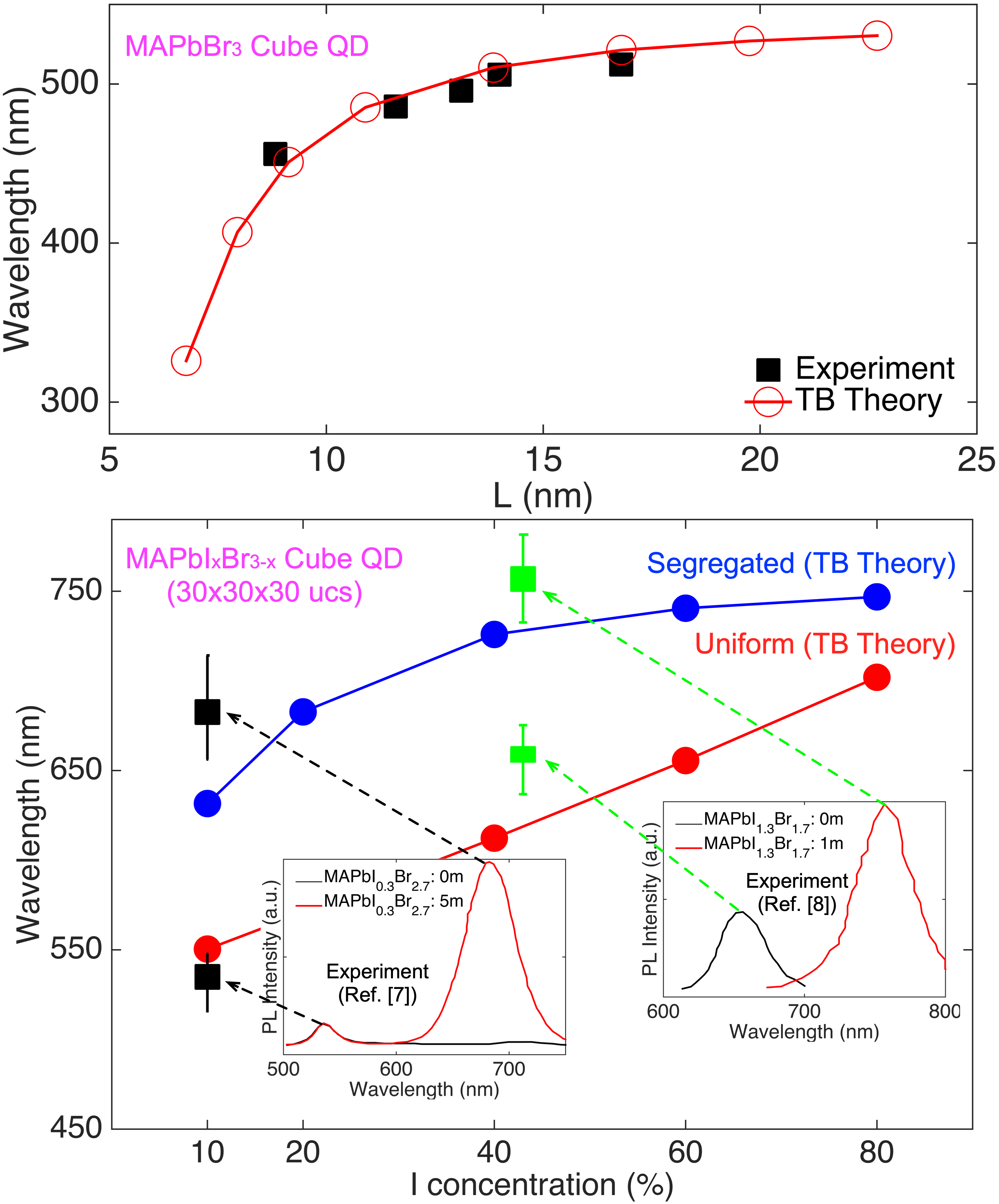The next connection between theory and experiment is established for bulk MAPbI$_{\mathrm{x}}$Br$_{\mathrm{3-x}}$ samples, where we used modeling results of cube QDs of the largest confinement size (30 ucs). The comparison is shown in the bottom sub figure of Fig. 5, where the point of analysis is the position of photoluminescence (PL) peaks. From the experimental data, it is clear that photoinduced phase separation moves the position of PL peaks to a longer wavelength in both samples that include 10% (MAPbI$_{\mathrm{0.3}}$Br$_{\mathrm{2.7}}$) (6) and 43% of I (MAPbI$_{\mathrm{1.2}}$Br$_{\mathrm{1.8}}$) in I-Br mixtures (7). The two positions of PL peaks measured for each sample are 535/683 nm (I= 10) and 656/757 nm (I=43%), and are marked with block and green boxes in the bottom subfigure of Fig. 5, where error bars indicate full widths at half maximum (FWHMs) of PL peaks. Modeling results here, shown with red and blue curves, also have nice accuracy so that the deviation calculated with respect to the median of experimental data is smaller than 50 nm (< 10% of experimental data) for the two composition ratios of interest. Note that this deviation becomes even smaller if FWHMs are considered.

## IV. CONCLUSIONS

Composition and size engineering of band gap energies are theoretically studied with tight-binding simulations for MAPbI$_{\mathrm{x}}$Br$_{\mathrm{3-x}}$ nanostructures of physically realizable sizes. With a map of theoretically achievable band gap energies that can be used an initial guideline for those who want to design materials aiming for a specific range of emission wavelengths, this work present strategies to overcome the red-shift problem induced by phase separation in halide mixtures, which serves as a critical bottleneck for stable light source designs.

### ACKNOWLEDGMENTS

This work has been supported by the Korea Institute of Science and Technology Information (KISTI) institutional R&D program (K-19-L02-C07), and the NURION high performance computing cluster has been extensively used for all the simulations.

### REFERENCES

1
Burschka J., Pellet N., Moon S., Humphry-Baker R., Gao P., Nazeeruddin M., Gratzel M., Jul 2013, Sequential Deposition as a Route to High-Performance Perovskite-Sensitized Solar Cells, Nature, Vol. 499, pp. 316-3192
Yang W., et al. , Jun 2017, Iodide management in formamidinium-lead-halide–based perovskite layers for efficient solar cells, Science, Vol. 30, pp. 1376-13793
Tan Z., et al. , Aug 2014, Bright Light-Emitting Diodes Based on Organometal Halide Perovskite, Nature Nanotechnology, Vol. 9, pp. 687-6924
Cho H., et al , Dec 2015, Overcoming the Electrolumine-scence Efficiency Limitations of Perovskite Light-Emitting Diodes, Science, Vol. 350, pp. 1222-12255
Cao Y., et al. , Oct 2018, Perovskite Light-Emitting Diodes Based on Spontaneously Formed Submicrometre-Scale Structures, Nature, Vol. 562, pp. 249-2536
Protesescu L., Yakunin S., Bodnarchuk M., Krieg F., Caputo R., Hendon C., Yang R., Walsh A., Kovalenko M., Jan 2015, Nanocrystals of Cesium Lead Halide Perovskites (CsPbX3, X = Cl, Br, and I): Novel Optoelectronic Materials Showing Bright Emission with Wide Color Gamut, Nano Letters, Vol. 15, pp. 3692-36967
Bischak C., Hetherington C., Wu H., Aloni S., Ogletree D., Limmer D., Ginsberg N., Jan 2017, Origin of Reversible Photoinduced Phase Separation in Hybrid Perovskites, Nano Letters, Vol. 17, pp. 1028-10338
Yoon S., Draguta S., Manser J., Sharia O., Schneider W., Kuno M., Kamat P., Jun 2016, Tracking Iodide and Bromide Ion Segregation in Mixed Halide Lead Perovskites during Photoirradiation, ACS Energy Letters, Vol. 1, pp. 290-2969
Kulkarni S., Baikie T., Boix P., Yantara N., Mathews N., Mhaisalkarab S., Mar 2014, Band-gap tuning of lead halide perovskites using a sequential deposition process, Journal of Materials Chemistry A, Vol. 2, pp. 9221-922510
Unger E., Kegelmann L., Suchan K., Sörell D., Korte L., Albrechtd S., Mar 2017, Roadmap and roadblocks for the band gap tunability of metal halide perovskites, Journal of Materials Chemistry A, Vol. 5, pp. 11401-1140911
Butler K., Frost J., Walsh A., Oct 2014, Band Alignment of the Hybrid Halide Perovskites CH3NH3PbCl3, CH3 NH3PbBr3 and CH3NH3PbI3, Materials Horizons, Vol. 2, pp. 228-23112
Mosconi E., Umari P., Angelisab F., Sep 2016, Electronic and optical properties of MAPbX3 perovskites (X = I, Br, Cl): A unified DFT and GW theoretical analysis, Physical Chemistry Chemical Physics, Vol. 18, pp. 27158-2716413
Even J., Pedesseau L., Katan C., Sep 2014, Understanding Quantum Confinement of Charge Carriers in Layered 2D Hybrid Perovskites, ChemPhysChem, Vol. 15, pp. 3733-374114
Jancu J., Scholz R., Beltram F., Bassani F., Mar 1998, Empirical spds* tight-binding calculation for cubic semiconductors: General method and material parameters, Physical Review B, Vol. 57, pp. 649315
Klimeck G., et al. , Aug 2007, Atomistic Simulation of Reali-stically Sized Nanodevices Using NEMO 3-D: PART I - Models and Benchmarks, IEEE Transactions on Electron Devices, Vol. 54, No. 9, pp. 2079-208916
Yao E., Yang Z., Meng L., Sun P., Dong S., Yang Y., Yang Y., Apr 2017, High-Brightness Blue and White LEDs based on Inorganic Perovskite Nano crystals and their Composites, Advanced Materials, Vol. 17, pp. 160685917
Draguta S., Sharia O., Yoon S., Brennan M., Morozov Y., Manser J., Kamat P., Schneider W., Kuno M., Aug 2017, Rationalizing the light-induced phase separation of mixed halide organic-inorganic perovs-kites, Nature Communications, Vol. 8, pp. 20018
Poglitsch A., Weber D., Aug 1987, Dynamic disorder in methylammoniumtrihalogenoplumbates (II) observed by millimeter-wave spectroscopy, Journal of Chem-ical Physics, Vol. 87, pp. 6373-637819
Noh J., Im S., Heo J., Mandal T., Seok S., Mar 2013, Chemical management for colorful, efficient, and stable inorganic-organic hybrid nanostructured solar cells, Nano Letters, Vol. 13, pp. 1764-176920
Boyer-Richard S., Katan C., Traore B., Scholz R., Jancu J., Even J., Sep 2016, Symmetry-Based Tight Binding Modeling of Halide Perovskite Semiconductors, Journal of Physical Chemistry Letters, Vol. 7, pp. 3833-384021
Ashhab S., Voznyy O., Hoogland S., Sargent E., Madjet M., Aug 2017, Effect of Disorder on Transport Properties in a Tight-Binding Model for Lead Halide Perovskites, Scientific Reports, Vol. 7, pp. 890222
Ryu H., Jeong Y., Kang J., Cho K., Aug 2016, Time-Efficient Simulations of Tight-binding Electronic Structures with Intel Xeon PhiTM Many-core Processors, Computer Physics Communications, Vol. 209, pp. 79-8723
Ryu H., Kwon O., Feb 2018, Fast, Energy-efficient Electronic Structure Simulations for Multi-million Atomic Systems with GPU Devices, Journal of Computational Electronics, Vol. 17, pp. 698-70624
KISTI Pushes the Boundaries of Science and Tech-nology with NURION, Intel® Case Study Report, https://www.intel.co.kr/content/www/kr/ko/products/docs/network-io/high-performance-fabrics/ opa-xeon-scalable-kisti-nurion-study.html25
NURION High Performance Computing Resource, https://www.top500.org/system/17942126
Jishi R., Ta O., Sharif A., Nov 2014, Modeling of Lead Halide Perovskites for Photovoltaic Applications, Journal of Physical Chemistry C, Vol. 118, pp. 28344-29349## Author

Hoon Ryu received B.S. degree from Seoul National University, Korea, M.S. degree from Stanford University, and Ph.D. degree from Purdue University, USA.

Dr. Ryu was with System LSI division in Samsung Electronics, and, is now a principal researcher at Korea Institute of Science and Technology Information, where he works nanoelectronics modeling for advanced materials and devices design with aids of numerical analysis.

His research interests include modeling studies on properties of semiconductor materials and devices, and performance optimization of large-scale numerical problems in high performance computers.